# AP Chemistry : Molar Mass

## Example Questions

### Example Question #1 : Molar Mass

Which of the following compounds has a formula weight within 74–75 g/mol?

BF3

KCl

C6H12O6

PCl5

KCl

Explanation:

PCl5, BF3, and C6H12O6 are not ionic compounds, and can thus be eliminated because "formula weight" implies that we are dealing with an ionic compound. This leaves only the correct answer, KCl, which can also be verified as correct by calculating the weight. K is 39g, while Cl is 35.5g. Together, this gives a total weight of approximately 74.5g.

### Example Question #1 : Molar Mass

The empirical formula of an unknown compound is CH2.  Which of the following could not be the molar mass of the unknown compound?

84.0 g/mol

70.0 g/mol

56.0 g/mol

42.0 g/mol

16.0 g/mol

16.0 g/mol

Explanation:

An empirical formula is a way of expressing a chemical formula in the smallest possible ratio. The compound C3H6 has an empirical formula of CH2 because the simplest ratio between this compound's constituent elements is one carbon for every two hydrogens.  This empirical formula can represent a number of compounds, but we know that each compound has a molar mass that is a multiple of the molar mass of the empirical formula. The empirical formula has a molar mass of 14 g/mol so the molar masses of compounds that can be represented by this empirical formula are (28,42,56 . . .).

### Example Question #3 : Molar Mass

What is the percent by mass of the anion in lithium iodate,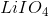?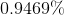This compound isn't ionic.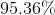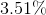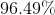Explanation:

Lithium iodate is comprised of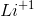and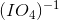. The anion refers to the negative ion, so we are looking for the percent by mass of.

The total molar mass of lithium iodate is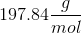.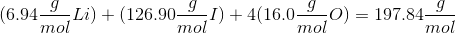The anion,, contributes 190.9g to the total mass.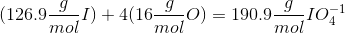Find the percent by mass by dividing the mass of the anion by the total molecular mass.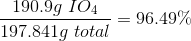### Example Question #4 : Molar Mass

What is the molar mass of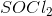?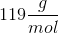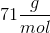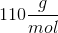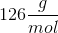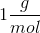Explanation:

To find the molecular weight, you need to add together the atomic masses of each of the atoms. 1 sulfur is 32g/mol, 1 oxygen is 16g/mol, and 2 chlorine is 71g/mol (each Cl atom is 35.5g/mol). These values are taken from the periodic table. Added together, they make 119g/mol.

### Example Question #1 : Molar Mass

What is the molar mass of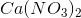?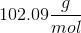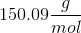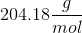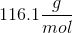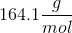Explanation:

The chemical formulahas a 2 right outside of the parentheses surrounding nitrate, so we need to distribute/multiply that 2 to everything inside the parentheses. That means that we have 1 calcium, two nitrogens, and six oxygens, and we need to add up all those masses to get the molar weight.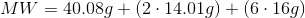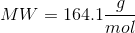### Example Question #6 : Molar Mass

What is the molar mass of potassium sulfide?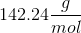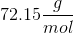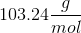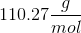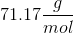Explanation:

In order to find the molar mass of potassium sulfide, you must first write out the chemical formula for potassium sulfide. The symbol for the potassium ion is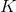, and the symbol for sulfur is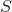. We know that this is an ionic compound because we have a metal involved (potassium) with a nonmetal (sulfur), so we must balance the net charge while making sure both atoms have a full octet. Since potassium is in group I, we know that it can easily lose one electron to fill its outer shell of electrons. Sulfur is in group VI, so we know that it can easily gain two electrons to fill its valence shell of electrons. It would take two potassium atoms to donate one electron each to sulfur to make this compound stable. Thus the chemical formula would be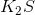. Use the periodic table to find the molar mass.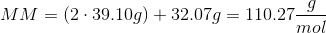### Example Question #1 : Molar Mass

What is the molar mass of ammonium hydroxide?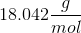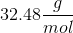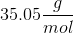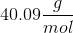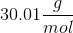Explanation:

The formula for ammonium hydroxide is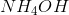. To find molar mass, add up the individual masses of each element, and remember to multiply that mass by the molar ratio of that element.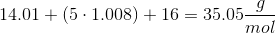### Example Question #51 : Stoichiometry

What is the molar mass of oxalic acid?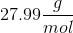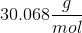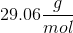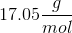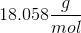Explanation:

First, write the formula for oxalic acid: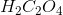. We know that this formula is correct because when we have an acid with an "-ic" ending and no "hydro-" prefix, that means it's coming from a polyatomic ion with an "-ate" ending. So we know that oxal"ic" acid is derived from the oxal"ate" polyatomic ion. The formula for that ion is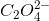. Putting a hydrogen atom in front of this ion, and balancing the charges, we get.Then add the atomic masses of each of the individual elements in their respective ratios, to get the molar mass for the whole compound.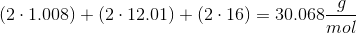### Example Question #1 : Molar Mass

A pure sample of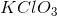is found to contain 71g of chlorine atoms. What is the mass of the sample?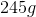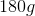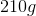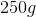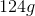Explanation:

First, find how many moles of chlorine are present in the sample: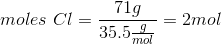Because the ratio of moles of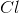to moles ofis 1:1, there are also 2 moles of. The next step is to find the mass of 2 moles of.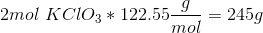### Example Question #1 : Molar Mass

A chemist has found that 2.0 moles of a mysterious compound contained 48g of carbon, 8g of hydrogen, and 64g of oxygen. What is the molecular formula of the compound?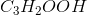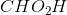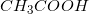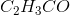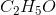Explanation:

Find the number of moles of each element.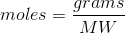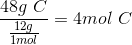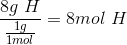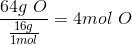If the chemist has 2.0 moles of the compound, 1 mole would contain half as much. So 1mol of carbon, 4mol of hydrogen, and 2mol of oxygen, which forms acetic acid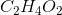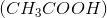### All AP Chemistry Resources